Math Calculators, Lessons and Formulas

It is time to solve your math problem

mathportal.org

# Operations with one complex number

This calculator extracts the square root, calculate the modulus, finds inverse, finds conjugate and transform complex number to polar form. The calculator will generate a step by step explanation for each operation.

Operations with one complex number
Five operations with a single complex number.
show help ↓↓ examples ↓↓
 conjugate (default) modulus inverse roots polar form
Find approximate solution
Hide steps
working...
examples
example 1:ex 1:
Find the complex conjugate of $z = \frac{2}{3} - 3i$.
example 2:ex 2:
Find the modulus of $z = \frac{1}{2} + \frac{3}{4}i$.
example 3:ex 3:
Find the inverse of complex number $3 - 3i$.
example 4:ex 4:
Find the polar form of complex number $z = \frac{1}{2} + 4i$.

## Formulas for conjugate, modulus, inverse, polar form and roots

### Conjugate

The conjugate of the complex number z = a + bi is: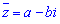Example 1: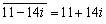Example 2: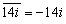Example 3: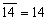### Modulus (absolute value)

The absolute value of the complex number z = a + bi is: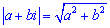Example 1: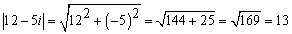Example 2: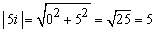Example 3: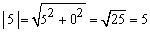### Inverse

The inverse of the complex number z = a + bi is: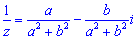Example 1: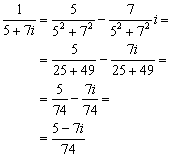Quick Calculator Search